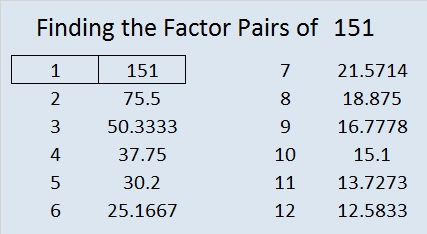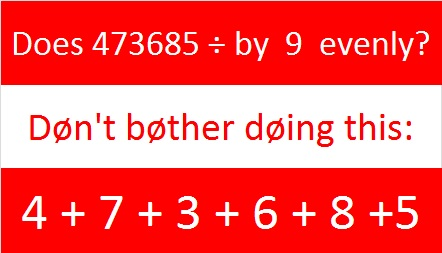# 151 and Why you shouldn’t even bother with that divisibility trick for 9 and 3

• 151 is a prime number.
• Prime factorization: 151 is prime.
• The exponent of prime number 151 is 1. Adding 1 to that exponent we get (1 + 1) = 2. Therefore 151 has exactly 2 factors.
• Factors of 151: 1, 151
• Factor pairs: 151 = 1 x 151
• 151 has no square factors that allow its square root to be simplified. √151 ≈ 12.2882How do we know that 151 is a prime number? If 151 were not a prime number, then it would be divisible by at least one prime number less than or equal to √151 ≈ 12.3. Since 151 cannot be divided evenly by 2, 3, 5, 7, or 11, we know that 151 is a prime number.

——————————————————————————————————————–I’m serious. Don’t bother using that trick that wants you to add up all the digits of a number to see if the number can be evenly divided by 3 or 9. Really, Don’t use it.

Even if it does take less time than actually dividing a whole number by 3 or by 9, it isn’t the best use of your time, and there are too many opportunities to make mistakes using it, especially if the whole number has more than a few digits.

One out of every three randomly chosen whole numbers will be divisible by 3. That’s pretty good odds if you guess, but I’m not advocating guessing.

I’m suggesting that you ignore most of the numbers and only add up a few them. Let me exaggerate the point I’m trying to make:  the following number is too big to put in your calculator, but would you really add up all its digits to see if it’s divisible by 3 or 9?

99999999999999999999997599999999999999999981999999999

No, you wouldn’t. You were probably able to tell that the number is divisible by 3 but not by 9 in seconds by ignoring most of the digits. What about this next number? It has the same digits but not in the same order. I’m sure you can tell almost as quickly that it is also divisible by 3 but not by 9.

99999999979999999999999599999999999999999991999999998

Yes, it’s true that the digits of most whole numbers aren’t mostly nines, but they can form small groups of numbers that are mostly multiples of nine. What are some common multiples of nine that you can ignore in any whole number? 0, 18, 27, 36, 45, 90, 234, 333, 567, 666, and even 1234567890. These digits can be ignored or eliminated no matter what order they appear in the whole number.

Let’s apply this process of elimination to a whole number that is too big for any standard calculator:Look at the number at the top of this article, 473685. All of the digits can be eliminated so it is divisible by both 3 and 9 (but not by 6 because the original number wasn’t even.) See how quick and easy it can be to use this process of elimination?

So the next time you need to determine if a whole number is divisible by 3, 6, or 9, try ignoring or eliminating as many digits as you can before you do any addition. It will save you so much time. With some of that time you save you could complete a puzzle such as this one whose clues are all divisible by 3.1.Joseph Nebus
•ivasallay